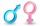Wood in the forest

The amount of wood in the forest was estimated at 6000 m3. How much wood will be in forest after 2 years if the annual growth of wood is 2.5% each year and logging 30 m3 each year?

Result

a =  6243 m3

Solution:Leave us a comment of example and its solution (i.e. if it is still somewhat unclear...):Math student
You ask for 2nd year but the answer is for the 5th...Dr Math
Yes, we corrected this problem, thank you !Next similar examples:

1. Highway repairThe highway repair was planned for 15 days. However, it was reduced by 30%. How many days did the repair of the highway last?
2. GP membersThe geometric sequence has 10 members. The last two members are 2 and -1. Which member is -1/16?
3. PercentsHow many percents is 900 greater than the number 750?
4. Geometric progression 2There is geometric sequence with a1=5.7 and quotient q=-2.5. Calculate a17.
5. SequenceFind the common ratio of the sequence -3, -1.5, -0.75, -0.375, -0.1875. Ratio write as decimal number rounded to tenth.
6. A perineumA perineum string is 10% shorter than its original string. The first string is 24, what is the 9th string or term?
7. Six termsFind the first six terms of the sequence a1 = -3, an = 2 * an-1
8. Theorem proveWe want to prove the sentence: If the natural number n is divisible by six, then n is divisible by three. From what assumption we started?
9. Test pointsIf you earned 80% of the possible 40 points, how many points did you miss to get 100%?
10. CardsSuppose that are three cards in the hats. One is red on both sides, one of which is black on both sides, and a third one side red and the second black. We are pulled out of a hat randomly one card and we see that one side of it is red. What is the probabi
11. Geometric progression 48,4√2,4,2√2
12. The ballThe ball was discounted by 10 percent and then again by 30 percent. How many percent of the original price is now?
13. The cityIn the city is 2/4 of women married to 3/4 men. What percentage of townspeople is single (not married)?
14. Percentages52 is what percent of 93?
15. CacaoCacao contains 34% filling. How many grams of filling are in 130 g cacao.
16. Base, percents, valueBase is 344084 which is 100 %. How many percent is 384177?
17. Apples 2James has 13 apples. He has 30 percent more apples than Sam. How many apples has Sam?